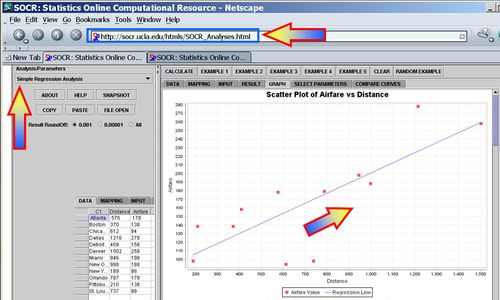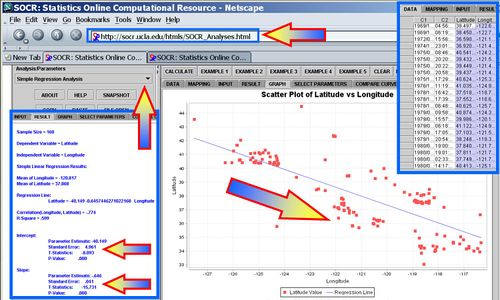# Difference between revisions of "AP Statistics Curriculum 2007 GLM Predict"

## General Advance-Placement (AP) Statistics Curriculum - Variation and Prediction Intervals

### Inference on Linear Models

Suppose we have again n pairs (X,Y), {$$X_1, X_2, X_3, \cdots, X_n$$} and {$$Y_1, Y_2, Y_3, \cdots, Y_n$$}, of observations of the same process. in the previous section, we discussed how to fit a line to the data. The main question is how to determine the best line?

#### Airfare Example

We can see from the scatterplot that greater distance is associated with higher airfare. In other words airports that tend to be further from Baltimore tend to be more expensive airfare. To decide on the best fitting line, we use the least-squares method to fit the least squares (regression) line.#### Confidence Interval Estimating of the Slope and Intercept of Linear Model

The parameters (a and b) of the linear regression line, $$Y = a + bX$$, are estimated using Least Squares. The least squares technique finds the line that minimizes the sum of the squares of the regression residuals, $$\hat{\varepsilon_i}=\hat{y}_{i}-y_i$$, $$\sum_{i=1}^N {\hat{\varepsilon_i}^2} = \sum_{i=1}^N (\hat{y}_{i}-y_i)^2$$, where $$y_i$$ and $$\hat{y}_{i}=a+bx_i$$ are the observed and the predicted values of Y for $$x_i$$, respectfully.

The minimization problem can be solved using calculus, by finding the first order partial derivatives and setting them equal to zero. The solution gives the slope and y-intercept of the regressions line:

• Regression line Slope:

$\hat{b} = \frac {\sum_{i=1}^{N} (x_{i} - \bar{x})(y_{i} - \bar{y}) } {\sum_{i=1}^{N} (x_{i} - \bar{x}) ^2}$ $\hat{b} = \frac {\sum_{i=1}^{N} {(x_{i}y_{i})} - N \bar{x} \bar{y}} {\sum_{i=1}^{N} (x_{i})^2 - N \bar{x}^2} = \rho_{X,Y} \frac {s_y}{s_x}$, where $$\rho_{X,Y}$$ is the correlation coefficient.

• Y-intercept:

$\hat{a} = \bar{y} - \hat{b} \bar{x}$

If the error terms are Normally distributed, the estimate of the slope coefficient has a normal distribution with mean equal to b and standard error given by:

$s_ \hat{b} = \sqrt { {1\over (N-2)} \frac {\sum_{i=1}^N \hat{\varepsilon_i}^2} {\sum_{i=1}^N (x_i - \bar{x})^2} }$.

$[ \hat{b} - s_ \hat{b} t_{(\alpha/2, N-2)},\hat{b} + s_ \hat{b} t_{(\alpha/2, N-2)}]$

In other words, if there is a 1 mile increase in distance the airfare will go up by between $0.054 and$0.180.

• Significance testing: If X is not useful for predicting Y this is like saying the true slope is zero. In a hypothesis test our status quo null hypothesis would be that there is no relationship between X and Y
Hypotheses$H_o: b = 0$ vs. $$H_1: b1 \not= 0$$ (or $$H_1: b > 0$$ or $$H_1: b < 0$$).
Test-statistics$t_o={b-0\over SE(b)}$, where $$t_o \sim t_{(df=n-2)}$$ is the T-Distribution.

#### Example

For the distance vs. airfare example, we can compute the standard error of the slope coefficient (b), SE(b) $SE(b)={37.83 \over \sqrt{1786499}}=0.0283$.

• Then a 95% confidence interval for b is given by:
CI(b)$b \pm t_{(\alpha/2, df=10)}SE(b)=0.11738 \pm 2.228\times 0.02832=[0.054 , 0.180].$
• Significance testing:

$t_o={b-0\over SE(b)}={0.11738-0 \over 0.02832}=4.145$ and $$p-value =0.002$$.

### Earthquake Example

Use the SOCR Earthquake Dataset to formulate and test a research hypothesis about the slope of the best-leaner fit between the Longitude and the Latitude of the California Earthquakes since 1900. You can see the SOCR Geomap of these Earthquakes. The image below shows how to use the Simple Linear regression in SOCR Analyses to calculate the regression line and make inference on the slope.